Author: Oscar Cronquist Article last updated on December 30, 2018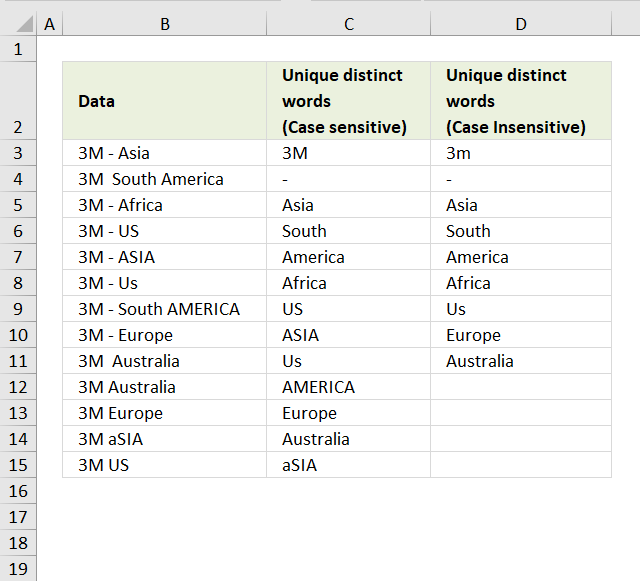This blog post describes how to create a list of unique distinct words from a cell range. Unique distinct words are all words but duplicate words are only listed once.

Cell range A2:A14 contains words, see picture below.

###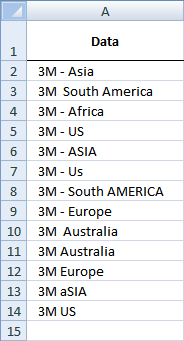Rick Rothstein (MVP - Excel) helped me out here with a powerful user defined function (udf).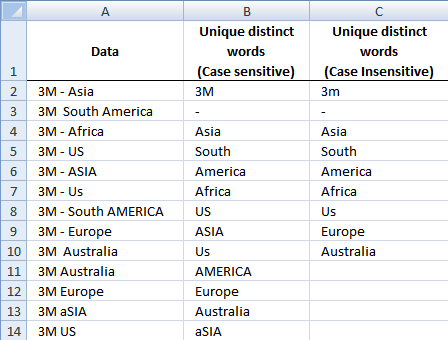Array formula in cell B2:B23

=ListOfWords(\$A\$2:\$A\$18, TRUE) + CTRL + SHIFT + ENTER

Select all the cells to be filled, then type the above formula  into the Formula Bar and press CTRL+SHIFT+ENTER

Array formula in cell C2:C23

=ListOfWords(\$A\$2:\$A\$18, FALSE) + CTRL + SHIFT + ENTER

Select all the cells to be filled, then type the above formula  into the Formula Bar and press CTRL+SHIFT+ENTER

### User defined function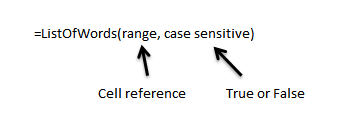Instructions

1. You can select far more cells to load the formulas in than are required by the list. The empty text string will be displayed for cells not having an entry.
2. You can specify a larger range than the there are filled in cells as the argument to these macros to allow for future entries in the column.
3. You can specify whether the listing is to be case sensitive or not via the optional second argument with the default value being FALSE, meaning duplicated entries with different casing like One, one, ONE, onE, etc.. will all be treated as if they were the same word with the same spelling. If you pass TRUE for that optional second argument, then those words would all be treated as if they were different words.
4. For all the "Case Insensitive" listing, the words are listed in Proper Case (first letter upper case, remaining letters lower case). The reason being if you had One, one and ONE then there is not reason to prefer one version over another, so I solved the problem by using Proper Case throughout.

VBA Code:

```Function ListOfWords(Rng As Range, Optional CaseSensitive As Boolean) As Variant
Dim X As Long, Index As Long, List As String, Words() As String, LoW As Variant
With WorksheetFunction
Words = Split(.Trim(Replace(Join(.Transpose(Rng)), Chr(160), " ")))
LoW = Split(Space(.Max(UBound(Words), Application.Caller.Count) + 1))
For X = 0 To UBound(Words)
If InStr(1, Chr(1) &amp;amp; List &amp;amp; Chr(1), Chr(1) &amp;amp; Words(X) &amp;amp; Chr(1), 1 - Abs(CaseSensitive)) = 0 Then
List = List &amp;amp; Chr(1) &amp;amp; Words(X)
If CaseSensitive Then
LoW(Index) = Words(X)
Else
LoW(Index) = StrConv(Words(X), vbProperCase)
End If
Index = Index + 1
End If
Next
ListOfWords = .Transpose(LoW)
End With
End Function
```

### How to copy above code to your workbook

1. Press Alt-F11 to open visual basic editor
2. Click Module on the Insert menu
3. Copy and paste above user defined function to code module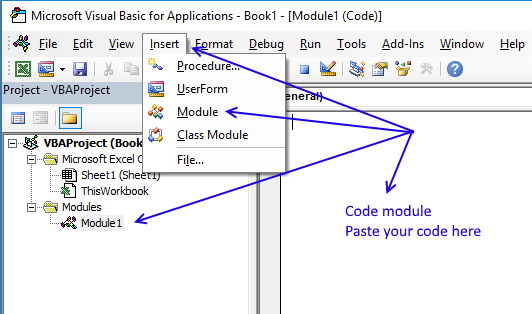4. Exit visual basic editor
5. Select a sheet
6. Select a cell range
7. Type =ListOfWords(\$A\$2:\$A\$18, TRUE) into formula bar and press CTRL+SHIFT+ENTER